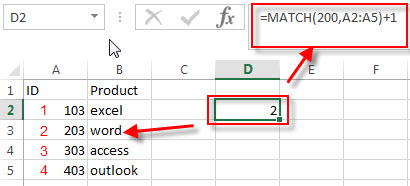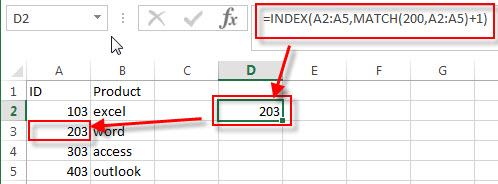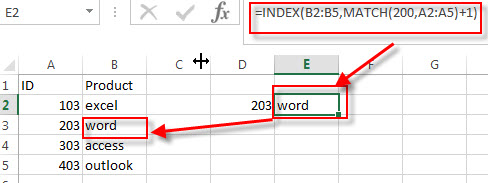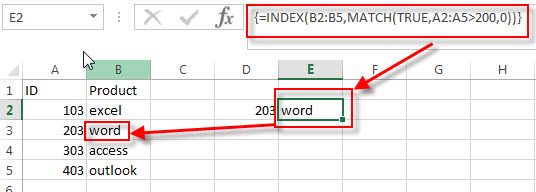How to Lookup the Next Largest Value

This post explains that how to lookup the next largest value in another column in excel. how to find the next largest value in a range with the excel formula.

Find the next largest value

If you want to get the next largest value in another column, you can use a combination of the INDEX function and the MATCH function to create an excel formula.

For example, if we used the VLOOKUP function to lookup the value 200, then it returns “103”, so it returns the value that is less than or equal to the lookup value. but if you want to return the next largest value in the range A2:A5, you can use the following formula:

=INDEX(A2:A5,MATCH(200,A2:A5)+1)

And if you want to find the next largest value in another column B2:B5, you just need to change the first argument of the INDEX function, use the range B2:B5 instead of A2:A5, the formula is as follwos:

=INDEX(B2:B5,MATCH(200,A2:A5)+1)

Let’s see how the above formula works:

=MATCH(200,A2:A5)+1The MATCH function return the position of the lookup value 200 in the range A2:A5, if the MATCH function is not find the exact match, then it would return an approximate matched value(it should be less than or equalt to the lookup value) by default, so it returns 1.

To get the position of the next largest value, the position number returned by the match function need to add 1 again. so now we got the position of the next largest value in the range A2:A5. It returns 2.

=INDEX(A2:A5,MATCH(200,A2:A5)+1)=INDEX(B2:B5,MATCH(200,A2:A5)+1)The INDEX function returns the value based on the given position that returned by the above MATCH function.

so if you used the Range A2:A5, then the above INDEX function returns the next largest value in the range A2:A5.

And if you used the Range B2:B5, then it returns the next largest value in the range B2:B5.

You can also use the following array formula to achieve the same result.

=INDEX(B2:B5,MATCH(TRUE,A2:A5>200,0))Note: when you enter the above array formula into a single cell, you should press Ctrl + Shift +Enter key.

Related Formulas

• Find the Relative Position in a Range or Table
If you want to know the relative row position for all rows in an Excel Range (B3:D6), you can use a excel Array formula as follows:=ROW(B3:D6)- ROW(B3) + 1. You can also use another excel array formula to get the same result as follows:=ROW(B3:D6)-ROW(INDEX(B3:D6,1,1))+1…
• Reverse a List or Range
If you want to reverse a list or range, you can use a combination of the INDEX function, the COUNTA function, the ROW function or ROWS function to create a new formula. you can use the following formula:=INDEX(\$A\$2:\$A\$5,COUNTA(\$A\$2:\$A\$5)-ROWS(\$C\$2:C2)+1)…

Related Functions

• Excel INDEX function
The Excel INDEX function returns a value from a table based on the index (row number and column number)The INDEX function is a build-in function in Microsoft Excel and it is categorized as a Lookup and Reference Function.The syntax of the INDEX function is as below:= INDEX (array, row_num,[column_num])…
• Excel MATCH function
The Excel MATCH function search a value in an array and returns the position of that item.The syntax of the MATCH function is as below:= MATCH  (lookup_value, lookup_array, [match_type])….
• Excel VLOOKUP function
The Excel VLOOKUP function lookup a value in the first column of the table and return the value in the same row based on index_num position.The syntax of the VLOOKUP function is as below:= VLOOKUP (lookup_value, table_array, column_index_num,[range_lookup])….
Related Posts

VLOOKUP Formula | Faster Trick with 2 VLOOKUPS

This post will guide you how to use 2 VLOOKUPS function to looking up data entries from a given range of cells in Microsoft Excel. VLOOKUP with 2 lookups can be faster than a single VLOOKUP in certain scenarios. The ...

VBA Macro For VLOOKUP From Another Sheet

In the previous post, you should know that how to fix or remove the #N/A error when using VLOOKUP formula to lookup value from another sheet. And this post will show you how to use VBA code to vlookup data ...

VLOOKUP From Another Sheet Not Working

In the previous post, you should know that how to fix or remove the #N/A error when using VLOOKUP formula to lookup value from another sheet. And this post will show you reasons why your VLOOKUP formula is not working ...

Fix #N/A Error For VLOOKUP From Another Sheet

This post will show you how to fix the #N/A error why it occurs when you extract values from another sheet using VLOOKUP function in Excel 2016,2013,2010 or other Excel versions. How can you correct a #N/A error in VLOOKUP ...

Get Employee Information by VLOOKUP

VLOOKUP is one of the key functions among all lookup & reference functions in Excel. Today, in this article, we will show you the way to apply VLOOKUP to retrieve employee information. I hope this article will help you in ...

VLOOKUP with Two Lookup Tables

VLOOKUP is one of the key functions among all lookup & reference functions in Excel. Today we will show you the application of VLOOKUP function when there are two lookup tables. EXAMPLE Table1 and table2 record the rates of Y2020 ...

VLOOKUP with Multiple Lookup Values

VLOOKUP is one of the key functions among all lookup & reference functions in Excel. It can scan and retrieve data from a static or dynamic table based on your lookup value. It can perform approximate match or exact match ...

VLOOKUP Data by Date

VLOOKUP is one of the key functions among all lookup & reference functions in Excel. It can scan and retrieve data from a static or dynamic table based on your lookup value. It can perform approximate match or exact match ...

VLOOKUP – Retrieve Data from Another Workbook

VLOOKUP is one of the key functions among all lookup & reference functions in Excel. It can scan and retrieve data from a static or dynamic table based on your lookup value. It can perform approximate match or exact match ...

VLOOKUP – Retrieve Data from Another Worksheet

VLOOKUP is one of the key functions among all lookup & reference functions in Excel. It can scan and retrieve data from a static or dynamic table based on your lookup value. It can perform approximate match or exact match ...

Sidebar